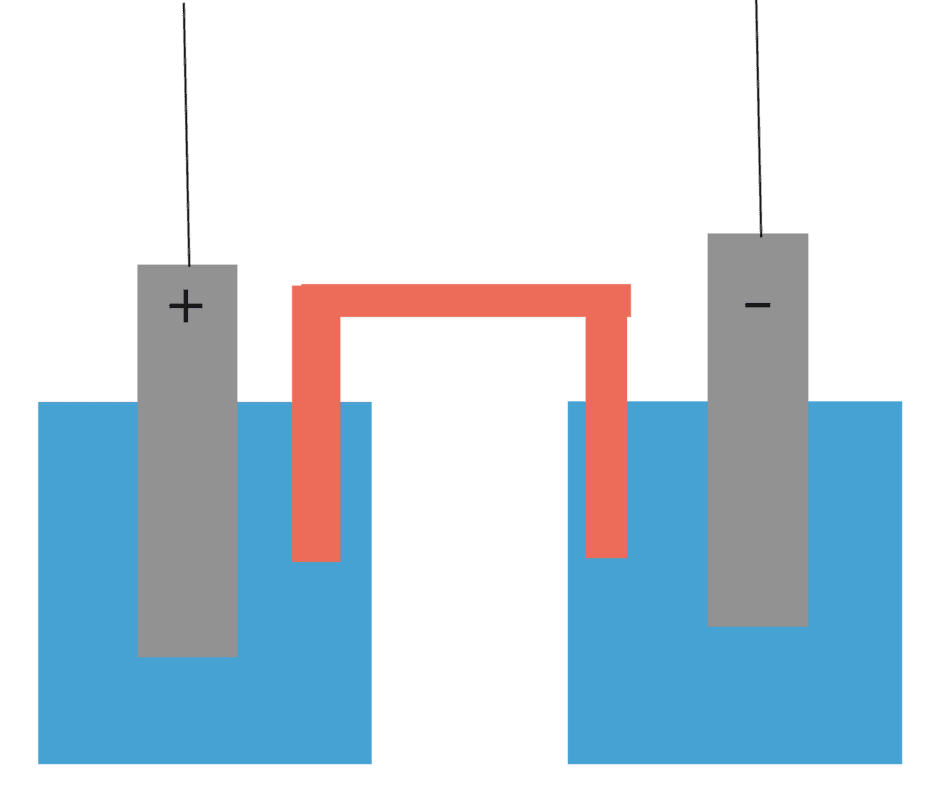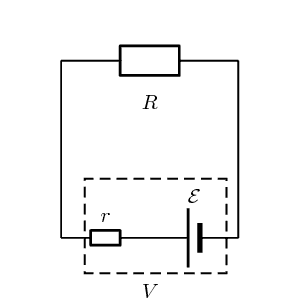# Putting internal resistance in series with a voltage source

• etotheipi

#### etotheipi

Suppose I have a galvanic cell, where I've arbitrarily set the (-) anode to have a potential of zero volts and the (+) cathode to ##\epsilon## V. The electrodes are connected via the load, but also via the solutions and salt bridge in the centre. Edit: The two trailing wires are connected to a load forming a complete circuit, though I haven't drawn them in.When idealised with a Thevenin equivalent in circuit theory, the internal resistance is put in series with the voltage source, and the current/voltage across the entirety of the practical cell can be modeled perfectly with this setup:However, looking at the physical cell, the internal resistance appears as if it is directly between the two electrodes, resulting from the electrolyte and salt bridge. If the potential difference between the electrodes has magnitude ##\epsilon##, then it sort of seems like the current through the cell should be ##\frac{\epsilon}{r}## if we apply Ohms law between the electrodes within the cell. Evidently this cannot be a correct approach, since from the unambiguous theoretical setup we know that ##I = \frac{\epsilon}{R+r}##.

I was wondering where the mistake occurs when applying Ohms law between the electrodes. Is it perhaps that the internal resistance arises elsewhere? Thank you!

Last edited by a moderator:
then it sort of seems like the current through the cell should be ϵrϵr\frac{\epsilon}{r}

I’m having trouble understanding your question. Visualizing the internal resistance as being “between the electrodes” or as a separate resistance in series with the cell doesn’t matter because they are in series. It is convenient for easy notation to show it as a separate resistance. I’m not sure how you would draw it between the electrodes, but if somebody wanted to make that symbol it would be fine, but it wouldn’t change how it works.

Your real question seems to be based on your notion that the current should be ##\frac {\epsilon} r ##, but how could that be? Current flows in a closed circuit. If the battery isn’t connected to a load, no current flows. For current to flow the battery must be connected to a load. When the battery is connected to a load the net load is the load plus the equivalent resistance of the battery. The equivalent resistance is never by itself.

•Dale
Your real question seems to be based on your notion that the current should be ##\frac {\epsilon} r ##, but how could that be? Current flows in a closed circuit. If the battery isn’t connected to a load, no current flows. For current to flow the battery must be connected to a load. When the battery is connected to a load the net load is the load plus the equivalent resistance of the battery. The equivalent resistance is never by itself.

I know this to be true, though consider a load attached to the two wires connected to the electrodes of the physical cell. A current flows. If the internal resistance ##r## lies between the electrodes actually within the cell, and the two electrodes are at potentials ##A## and ##B##, then the current between them should be by Ohm's law ##\frac{A-B}{r}##. It seems no different to having a resistor with one side at ##6##V and the other at ##2##V, and working out the current through it to be ##\frac{6-2}{R}##.

current through the cell should be ϵrϵr\frac{\epsilon}{r} if
That would be the short circuit current.

The voltage of the cell is taken as the open circuit voltage, with "no" current flowing.

If one adds a load R, then of course the same current flows through R and r.

That would be the short circuit current.
The voltage of the cell is taken as the open circuit voltage, with "no" current flowing.
If one adds a load R, then of course the same current flows through R and r.

I know what you are referring to, though I'm thinking about the first galvanic cell example. Even in a steady state, if the two electrodes have a pd. of ##\epsilon## calculated from the standard reduction potentials, then I'm wondering what the mistake is in applying Ohms law along the section of circuit from one electrode, across the salt bridge and to the other electrode is, taking this section of circuit to be the internal resistance (if it happens to be so - I'm not sure!).

If we write the cell as a Thevenin equivalent then everything works out fine, using normal circuit theory rules, since the ideal voltage source symbol itself has no resistance.

•256bits
The ir drop within the cell reduces the potential between the output electrodes of the cell.
The resistor R sees less of a voltage than if there was no internal resistance.

Equivalents are not the reality, they are equivalent to the reality within some limits of validity.
Therefore, you are overstretching the equivalent to try to visualize the underlying physics.

Ohm's law doesn't apply inside the cell.

Even Ohm's law is not necessarily true and accurate in all cases. The relationship between voltage and current can be nonlinear.

Some people make the analogy that current in a wire is the equivalent to water flowing in a pipe. You wouldn't use that to infer that electrons are wet.

Use critical thinking. Most things you will learn in science and engineering are correct in most cases but not in all cases.

•Dale and etotheipi
Even Ohm's law is not necessarily true and accurate in all cases. The relationship between voltage and current can be nonlinear.

Ohm my, I should have considered this! Thank you.

That would be the short circuit current.

The voltage of the cell is taken as the open circuit voltage, with "no" current flowing.

If one adds a load R, then of course the same current flows through R and r.
Indeed, and that's why the "load resistance" is in series with the "internal resistance" of the battery, which answers the question in the OP.

Is it perhaps that the internal resistance arises elsewhere?
You are thinking of the internal resistance too physically. The internal resistance is a model, not a specific physical item. Basically, if you plot the current vs voltage curve for a battery you find that at 0 amps it has a certain voltage and that voltage decreases as the current increases. Adding an internal resistance term gives a first approximation to this decrease in voltage. It is not an exact description of the voltage-current relationship, but simply a good first order model.

••vanhees71 and etotheipi
Ohm my, I should have considered this! Thank you.
I see what you did there...•etotheipi